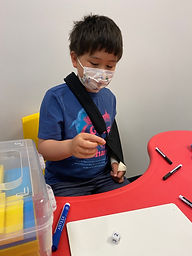## Ms. Briana

### Target 1​

###### Lesson Type:

Continuation

Number Operation

:

Computation

Multiply within 100.

###### 1:

Understand that multiplying numbers makes numbers larger.

###### 2:

Understand that multiplication equations can be written both vertically and horizontally and multiplication is often identified by an “x” in an equation.

###### 3:

Understand that multiplication represents the combination of equal groups of objects.

###### 4:

Understand that the same process is utilized to multiply 3 or more whole numbers that is used to multiply only two numbers (i.e., 2x3x4 = 2 groups of 3 in 6, and 4 groups of 6 is 24).

###### 5:

Understand that in multiplication equations, the first factor represents the number of groups and the second factor represents the quantity in each group.

2nd

###### Vocabulary:

Vertically, Horizontal, Combination, Multiplication, Arrays and Skip Counting

Activities:

Students used the small number spinners and 6 sided dice to create multiplication equations.

Students used white boards to practice repeated eddition method of multipliction solving. (example problem on the board with steps labeled then slowly we erased steps)### Home Exploration

###### Guiding Questions:## Absent Students:

### Target 2

:

###### 1:

Use a number to represent base ten block quantities.

###### 2:

Represent the composition or decomposition of three-digit numbers with an addition equation (113 = 100+10+3 or 1 hundred 1 ten and 3 ones).

###### 3:

Represent a multi-digit number in expanded format.

###### 4:

Understand how to “regroup” based on place-value understandings (i.e., ten ones can be composed into one ten – ten tens can be composed into one hundred).

###### 5:

Use place-value understandings to create numbers within specific limits.

2nd

###### Vocabulary:

Expanded Form, Place-Value, Regrouping, Composition and Decomposition

Activities:

Students took turns using the large spinner to create multi-digit quantities.

Students then expanded the quantities that they created with the spinner.### Home Exploration

###### Guiding Questions:### Target 3

:

###### 2:

Identify subtrahends and differences.

###### 3:

Identify multiplicands, multipliers, factors, and products.

###### 4:

Identify dividends, divisors, and quotients.

2nd

###### Vocabulary:

Addens, Sum, Subrtahends, Differences, Multiplicands, Mulipliers, Factors, Products, Dividends, Divisors and Quotients

Activities:

Students used playing cards to create different types of equations.

Students then took turns coming up to the large white board and naming different aspects of the equations correctly. (Played as a game and a race to see who could make it to the front first)### Home Exploration